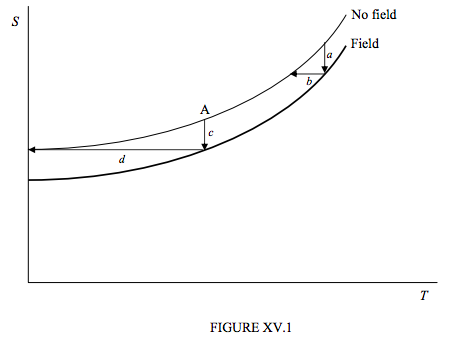# 15.4: Entropy and Temperature

$$\newcommand{\vecs}{\overset { \rightharpoonup} {\mathbf{#1}} }$$ $$\newcommand{\vecd}{\overset{-\!-\!\rightharpoonup}{\vphantom{a}\smash {#1}}}$$$$\newcommand{\id}{\mathrm{id}}$$ $$\newcommand{\Span}{\mathrm{span}}$$ $$\newcommand{\kernel}{\mathrm{null}\,}$$ $$\newcommand{\range}{\mathrm{range}\,}$$ $$\newcommand{\RealPart}{\mathrm{Re}}$$ $$\newcommand{\ImaginaryPart}{\mathrm{Im}}$$ $$\newcommand{\Argument}{\mathrm{Arg}}$$ $$\newcommand{\norm}{\| #1 \|}$$ $$\newcommand{\inner}{\langle #1, #2 \rangle}$$ $$\newcommand{\Span}{\mathrm{span}}$$ $$\newcommand{\id}{\mathrm{id}}$$ $$\newcommand{\Span}{\mathrm{span}}$$ $$\newcommand{\kernel}{\mathrm{null}\,}$$ $$\newcommand{\range}{\mathrm{range}\,}$$ $$\newcommand{\RealPart}{\mathrm{Re}}$$ $$\newcommand{\ImaginaryPart}{\mathrm{Im}}$$ $$\newcommand{\Argument}{\mathrm{Arg}}$$ $$\newcommand{\norm}{\| #1 \|}$$ $$\newcommand{\inner}{\langle #1, #2 \rangle}$$ $$\newcommand{\Span}{\mathrm{span}}$$$$\newcommand{\AA}{\unicode[.8,0]{x212B}}$$

Cooling by adiabatic demagnetization involves successive isothermal magnetizations followed by adiabatic demagnetizations, and this suggests that some insight into the process might be obtained by following it on an entropy : temperature (S : T) diagram.In figure XV.1 I draw schematically with a thin curve the variation of entropy of the specimen with temperature in the absence of a magnetizing field, and, with a thick curve, the (lesser) entropy of the more ordered state in the presence of a magnetizing field. The process a represents an isothermal magnetization, and the process b is the following adiabatic (isentropic) demagnetization, and it is readily seen how this results in a lowering of the temperature.

By the time when we reach the point A, an isothermal magnetization is represented by the process c, and the following adiabatic demagnetization is the process d, which takes us down to the absolute zero of temperature.

We shall find out in the next Chapter, however, that there is a fundamental flaw in this last argument, and that getting down to absolute zero isn’t going to be quite so easy.

This page titled 15.4: Entropy and Temperature is shared under a CC BY-NC license and was authored, remixed, and/or curated by Jeremy Tatum.